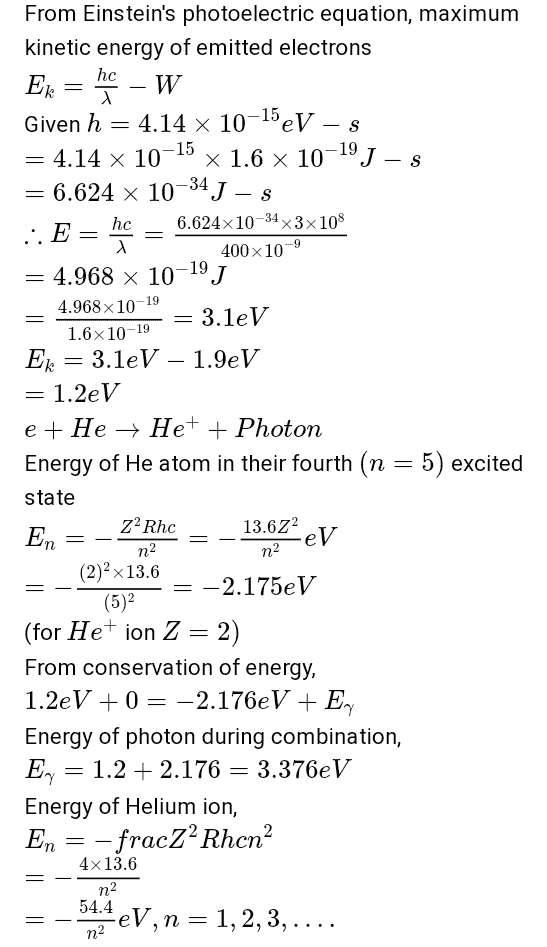22 views

Photoelectrons are emitted when 400 nm radiation is incident on a surface of work function 1.9eV. These photoelectrons pass through a region containing α-particles. A maximum energy electron combines with an α-particle to form a He+ ion, emitting a single photon in this process. He+ ions thus formed are in their fourth excited state. Find the energies in eV of the photons, lying in the 2 to 4eV range, that are likely to be emitted during and after the combination. [Take , h = 4.14  x  10-15 eV -s ]

in Physics | 22 views$$=-54.4 eV, -13.6 eV, -6.04 eV, -3.4 eV, -2.176 ev, -1.51 eV$$

Difference of energies lying between 2 and 4 eV is

$$-3.4+6.04=2.64 eV$$

$$-2.176+6.04=3.86 eV$$

Energies of photons emitted are 2.64 eV and 3.86 eV

by Expert (10.9k points)